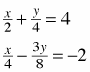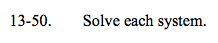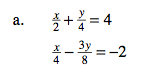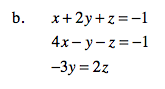### Home > A2C > Chapter Ch13 > Lesson 13.1.3 > Problem13-50

13-50.
1. Solve each system. Homework Help ✎

1.2. x + 2y + z = −1
4xyz = −1
−3y = 2zEliminate the denominators by
multiplying before trying to solve.

2x + y = 16

2x − 3y = −16

(4, 8)Solve the last equation for z in terms of y,
then substitute into the other two equations
to find x and y. Use those values to find z.